# C & C++

Showing page: 1 of 1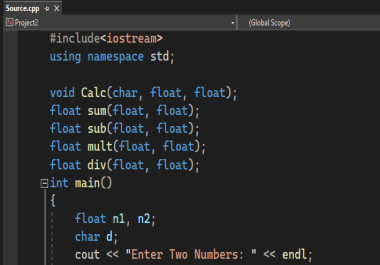BASIC CALCULATOR from Programming Fundamentals in C Pure C Code. Use...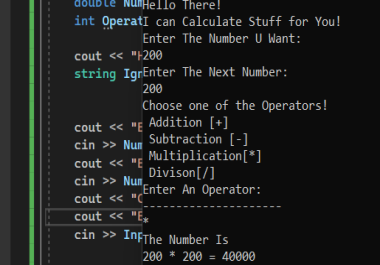The Calculator. It can do Addition, Subtraction, Multiplying and Div...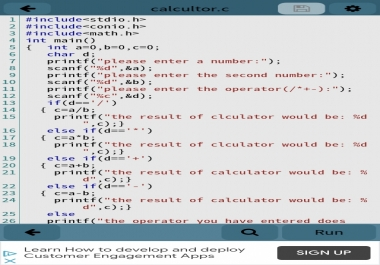Hello guys.I wanted to introduce my application that is a simple cal...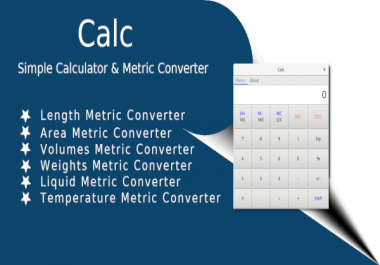Calc - simple calculator with metric converterbeside its simple calc...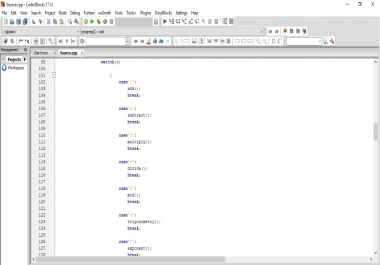No! this is not a pornography related piece of Code. This is a calcu...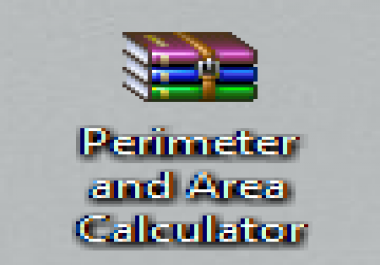This calculator can calculate perimeter and area of square, rectangl...